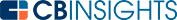# What is the Marginal Revenue Formula?Marginal revenue is the revenue generated by selling one additional unit. It equals the change in total revenue divided by the change in quantity.

The formula for calculating marginal revenue is:

The formula can also be represented in the following way:## How is marginal revenue different from total revenue?

Marginal revenue measures the revenue change from selling one additional unit. Total revenue measures the revenue generated by all the units sold. You can calculate it by multiplying price by quantity.

## What is an example of a marginal revenue calculation?

A candy manufacturer sells 5 pieces of chocolate for \$2.00 each. Total revenue equals \$10.00. If 6 pieces of chocolate sell for \$1.90 each, the new total revenue equals \$11.40. The change in total revenue is \$1.40. The change in quantity of chocolate is 1. Take the change in total revenue (\$1.40) divided by the change in quantity (1) to equal a marginal revenue of \$1.40.

## What is the relationship between marginal revenue and marginal cost?

Marginal revenue and marginal cost are measured by companies to determine profitability. If marginal revenue is greater than marginal cost, a company is profitable. If they are equal, the company’s profit is at equilibrium. If marginal cost is greater than marginal revenue, the company is operating at a loss.

To demonstrate this principle, let’s look at an example. A corn manufacturer can harvest its corn from 3 different fields. One field is easy to navigate, and the marginal cost of harvesting 1 stalk of corn is \$0.05. The second field is a little more difficult to navigate, and the marginal cost of harvesting 1 stalk of corn here is \$0.07.

A third field is treacherous and requires more heavy machinery. The marginal cost of harvesting 1 stalk of corn here is \$0.15. The corn manufacturer sells its corn for 12 cents per stalk. The profit earned from harvesting corn in the first field is 7 cents (\$0.12 marginal revenue - \$0.05 marginal cost).

The profit earned from harvesting corn in the second field is \$0.055 cents (\$0.12 marginal revenue - \$0.07 marginal cost). If the corn manufacturer used the third field, the corn harvested from here would result in a loss of \$0.03 (\$0.12 marginal revenue - \$0.15 marginal cost).

Therefore, it decides to harvest and sell corn only from fields one and two. Using marginal revenue and marginal cost to determine profitability, it concludes that harvesting corn from the third field is not worth the trouble. Companies can maximize profits by calculating marginal cost and marginal revenue and understanding the relationship between the two.

Marginal revenue is the change in revenue from selling 1 additional unit. It can be calculated by dividing the change in total revenue by the change in quantity.

Total revenue is different from marginal revenue because it measures the revenue generated from all units sold. Companies use the difference between marginal revenue and marginal cost to measure profitability.

You may also like# www.cbinsights.com